Objects and Methods : Reservoir Methods : User Methods : Regulated Spill Overflow
Regulated Spill Overflow
The category, Regulated Spill Overflow, is added if one of the following regulated spill methods is selected:
• Regulated Spill
• Regulated and Unregulated
• Regulated and Bypass
• Regulated, Bypass and Unregulated
• Bypass, Regulated and UnregulatedNone
This is the default, no-action method.Closed Gate Overflow
This method models the uncontrolled flow over a closed regulated spill gate. This functionality uses the Regulated Spill Capacity Fraction to compute the default amount of spillway that is overtopped.
This functionality only applies to Regulated Spill, not Bypass.
Slots Specific to This Method
Closed Gate Overflow
Type: Series Slot
Units: Flow
Description: Uncontrolled portion of the Regulated spill that overtops the gates.
Information: This value is computed by the regulated spill method as the value found on the Closed Gate Overflow Table multiplied by the Closed Gate Overflow Capacity Fraction
I/O: Output only
Closed Gate Overflow Table
Type: Table Slot
Units: Length vs Flow
Description: Pool Elevation vs unregulated flow.
Information: This table is used to specify the rating curve for uncontrolled flow over the closed Regulated Spill gates. The values should be input to the table as though every Regulated Spill gate is closed. The tables would start with zero flow at or just below the top of the closed gates.
I/O: Input only
Closed Gate Overflow Capacity Fraction
Type: Series Slot
Units: Fraction
Description: The fraction of the closed gate overflow that is available.
Information: The value must be between 0.0 and 1.0, inclusive. If not input or set by a rule, it defaults to (1 - Regulated Spill Capacity Fraction). Example: if 1 of 8 gates are unavailable, the Regulated Spill Capacity Fraction would be set to 0.875 and the Closed Gate Overflow Capacity Fraction would default to 0.125.
I/O: Input, set by a rule, or output
Method Details
If not input or set by a rule, Closed Gate Overflow Capacity Fraction defaults to (1 ‑ Regulated Spill Capacity Fraction). This default indicates that the overflow only happens over gates that are closed. Otherwise, the user can specify the Closed Gate Overflow Capacity Fraction slot to say how much of the overflow structure is available.
When the reservoir is below the top of the gates, there is no Closed Gate Overflow. But once the reservoir is above the top of one or more closed gates, there is Closed Gate Overflow. The computation of this overflow is similar to the unregulated spill computation.
1. A temporary variable called “initHW” is created to represent the Pool Elevation at the beginning of the timestep. Likewise, “endHW” is created to represent the Pool Elevation at the end of the timestep (If the Pool Elevation at the end of the timestep is not known, endHW is set equal to the Pool Elevation at the beginning of the timestep.)
2. The “Closed Gate Overflow Crest” is found from the Closed Gate Overflow Table. It is the Pool Elevation that corresponds to an overflow of zero.
3. If both initHW and endHW are less than or equal to the Closed Gate Overflow Crest, Closed Gate Overflow is set equal to zero.
4. If both initHW and endHW are greater than the Closed Gate Overflow Crest, the average Pool Elevation is used to determine the Closed Gate Overflow from the Closed Gate Overflow Table.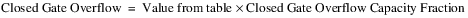5. If either initHW or endHW is greater than the Closed Gate Overflow Crest and the other is lower than the crest, the following evaluations and computations are performed: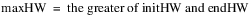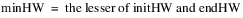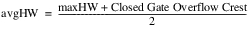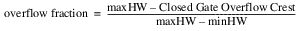where:
– maxHW = the maximum value of Pool Elevation over the timestep.
– minHW = the minimum value of Pool Elevation over the timestep.
– avgHW = the average Pool Elevation causing overflow over the timestep.
– overflow fraction = corresponds to the fraction of the timestep during which overflow occurs.
A temporary variable called “temp overflow” is obtained from the linear interpolation of the Closed Gate Overflow Table using avgHW. Closed Gate Overflow is then calculated as follows: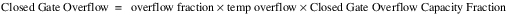When allocating spills to various structures, the Closed Gate Overflow must occur at the same time as unregulated spills; that is, before regulated or bypass. Then any remaining outflow can go through the regulated and or bypass spill structures. Therefore, the minimum regulated spill is computed as follows: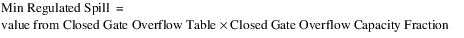Also, the Closed Gate Overflow is set equal to the Min Regulated Spill.
The functionality assumes that water is either flowing through the gate or over topping it, but not both. The method assumes that the Gate Overflow Table is fixed, that is the elevations in the table do not change. Thus if you had a gate stuck with 1/2 ft open at the bottom and there was still water going over the top, the table (which assumes the gate is closed) would be an incorrect rating.
Revised: 01/11/2023